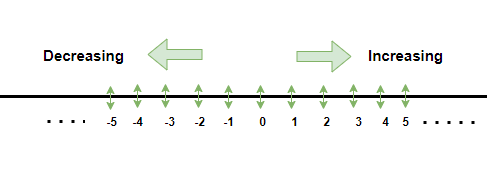Open in App
Not now

# Is Every Whole Number a Natural Number?

• Difficulty Level : Easy
• Last Updated : 21 Jul, 2021

Number system is the most basic pictorial representation of numbers on a number line, it is drawn by first making a straight line and then making negative, zero, and positive integers on the line. The negative numbers lie on the left side of zero and the positive numbers lie on the right side of zero. On moving to the right side, the value of the integers keeps on increasing systematically and on moving to the left side, value of the integers decrease.

### Integers, Whole numbers and Natural numbers

Integers, whole numbers and natural numbers are different terms, but they all can be correlated to each other in some way, lets look at the in depth meaning of these terms,

Integers

Integers are the numbers written and shown on the number line, all the numbers, positive, negative, even zero fall under the category of Integers. Any number that is written in fractional form will not be considered as an Integer. The meaning of this latin word is “Whole” and therefore, the number must be complete within itself. The number line is the best way to represent Integers,Whole Numbers

Whole numbers are represent as “W”, these are defined as the numbers that generate from 0 and goes upto infinity. Hence, it can be said that all the positive numbers including the number 0 are whole numbers. Two whole numbers when added to each other will give a whole number, however if 0 is added to another whole number, it will give the latter number and the number 0 has no effect and hence, is called as an identity number.

Natural Numbers

Natural numbers are represented as “N”, these are defined as the numbers that generate from 1 and goes upto infinity. Hence, 0 is not included in the Natural numbers, and it can be said that all positive integers are Natural numbers.

N = {W}- 0

### Is every Whole Number a Natural number?

The answer to the above question is simple. NO, every whole number is not a natural number. There is a very small difference between the definition of a whole number and a natural number and due to that it is not possible to conclude that every whole number is a natural number.

Whole numbers are all positive numbers including zero, but natural numbers do not contain zero and therefore, if 0 is removed from the Whole numbers, in that case, it can be equal to all natural numbers.

N = {W} – 1

However, if the question was framed in the reversed manner, that is, if every natural number is a whole number or not? Then the answer would be yes. Since, all natural numbers are whole numbers but not Vice- versa.

My Personal Notes arrow_drop_up